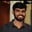Related Tags

communitycreator

# What are parse trees and different types of derivation?Buchiredddypalli Koushik

### Overview

Parse trees hierarchically represent the terminals and non-terminals. They generate input strings from the given grammar.

In the theory of computation, grammar is a finite set of formal rules that generate syntactically correct sentences.

Formally, grammar is defined as four tuples. In G=(V, T, P, S), G is the grammar consisting of a production rules’ set. It generates the strings of a language.

A terminal is a symbol that does not appear on the left-hand side of any production. Terminals are generally represented by small case letters.

Terminal symbols are components of the sentences generated using a grammar, for example, a, b, +, ( ,d.

A non-terminal is a non-leaf node in a parse tree. Non-terminals are represented by upper case letters.

Non-terminal symbols take part in sentence generation but are not the sentence’s component, for example, A, D, S.

### Rules for drawing a parse tree

• The root node is the starting symbol of the grammar.

• All leaf nodes or the end nodes present in the tree must be terminal.

• All internal nodes present in the tree must be non-terminal.

We can derive a parse tree in two ways:

• Leftmost derivation
• Rightmost derivation

Leftmost Derivation: This is the process of deriving the input string by expanding the leftmost non-terminal.

We try to derive the string in the leftmost derivation by changing the leftmost non-terminal to get the input string.

### Example

S → aS / ∈

For generating strings ‘aaa’.

S → aS

Note: Here, we try to add production rules to the leftmost non-terminal (i.e., S). This continues for the next steps as well.

→ aaS (Using S → aS)

→ aaaS (Using S → aS)

→ aaa∈ (Using S → ∈)

→ aaa

In the example above, we try to change the leftmost non-terminal to get the desired output everytime.

Leftmost Derivation

We expand the tree using the leftmost derivation in the diagram above.

First, we expand S, the leftmost non-terminal in the given example. Then, we expand the tree in the same fashion by first reducing the leftmost non-terminal to get the given input string.

Therefore, in the tree above, we expand the nodes from the leftmost non-terminal and successfully get the given input string.

Rightmost Derivation: This is the process of deriving the input string by expanding the rightmost non-terminal.

In the rightmost derivation, we derive the string by changing the rightmost non-terminal to get the input string.

### Example

S → aSS / ∈

For generating strings ‘aaa’.

S →aSS

Note: Here, we try to add production rules to the rightmost non-terminal(i.e., S). This continues for the next steps as well.

We try to change the S in the example everytime because it is the rightmost non-terminal.

→aSS (Using S → aS)

→aSaS (Using S → aS)

→aSaaS (Using S → aS)

→aSaaS (Using S → ∈)

→aSaa (Using S → ∈)

→aaa (Using S → ∈)

In the example above, we try to change the rightmost non-terminal to get the desired output.

In the diagram, we expand the tree using the rightmost derivation.

First, we expand the rightmost non-terminal, S, in the given example. Then, we expand the tree in the same fashion by first reducing the rightmost non-terminal to get the given input string.

Therefore, in the tree above, we expand the nodes from the rightmost non-terminal and successfully get the given input string.

RELATED TAGS

communitycreator

CONTRIBUTORBuchiredddypalli Koushik
RELATED COURSES

View all Courses

Keep Exploring

Learn in-demand tech skills in half the time